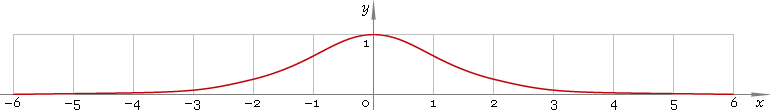The Art of Interface

# sech — hyperbolic secant function

Category. Mathematics.

Abstract. Hyperbolic secant: definition, plot, properties and identities.

## 1. Definition

Hyperbolic secant is defined as

sechx ≡ 2 /(ex + ex)

## 2. Graph

Hyperbolic secant is symmetric function defined everywhere on real axis. Its plot is depicted below — fig. 1.Fig. 1. Plot of the hyperbolic secant function y = sechx.

Function codomain is limited to the range (0, 1].

## 3. Identities

Base:

sech2x + tanh2x = 1

By definition:

sechx ≡ 1 /coshx

Property of symmetry:

sech−x = sechx

## 4. Support

Hyperbolic secant function sech of the real argument is supported by free version of the Librow calculator.

Hyperbolic secant function sech of the complex argument is supported by professional version of the Librow calculator.

## 5. How to use

To calculate hyperbolic secant of the number:

``sech(-1);``

To calculate hyperbolic secant of the current result:

``sech(rslt);``

To calculate hyperbolic secant of the number x in memory:

``sech(mem[x]);``